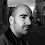## Friday, February 10, 2012

### New version of phyl.RMA for reduced major axis regression

I just posted a new version of phyl.RMA which also computes r2, T, df, and a P value for the test. The r2 is just the squared phylogenetic correlation. The other values are based on McArdle (1987). Direct link to code is here.

By default, the function tests the null hypothesis that the RMA slope is equal to 1.0. It is possible to change this default; however it is generally not possible to test for β=0.0 as this would only be true if σY2 was zero.

Here's a quick illustration of how it works, using functions in the geiger package to generate data under the null hypothesis for β (that is, β=1.0), but with λ=0.7:

> require(phytools)
> require(geiger)
> source("phyl.RMA.R")
> tree<-pbtree(n=150)
> X<-sim.char(lambdaTree(tree,0.7), model.matrix=matrix(c(2,1.5,1.5,2),2,2))[,,1]
> rma<-phyl.RMA(tree=tree,X[,1],X[,2],method="lambda")
> rma
\$RMA.beta
 0.1901904 1.0659599
\$V
x        y
x 2.305487 1.913151
y 1.913151 2.619657
\$lambda
 0.7334247
\$logL
 -604.2649
\$test
r2           T          df           P
0.6060265   1.2380363 115.5828809   0.2182114
\$resid
[,1]
t1   -1.543336686
t2    0.250290514
t3   -1.147190156
t4   ...

1.Is there a way to get SE or confidence intervals for phyl.RMA slopes?

1.Hi Anna.

Not sure, but many statisticians recoil at the idea of using the RMA regression at all, and especially not for hypothesis testing.... What are you hoping to do with it?

All the best, Liam

2.Hi Liam,

3.